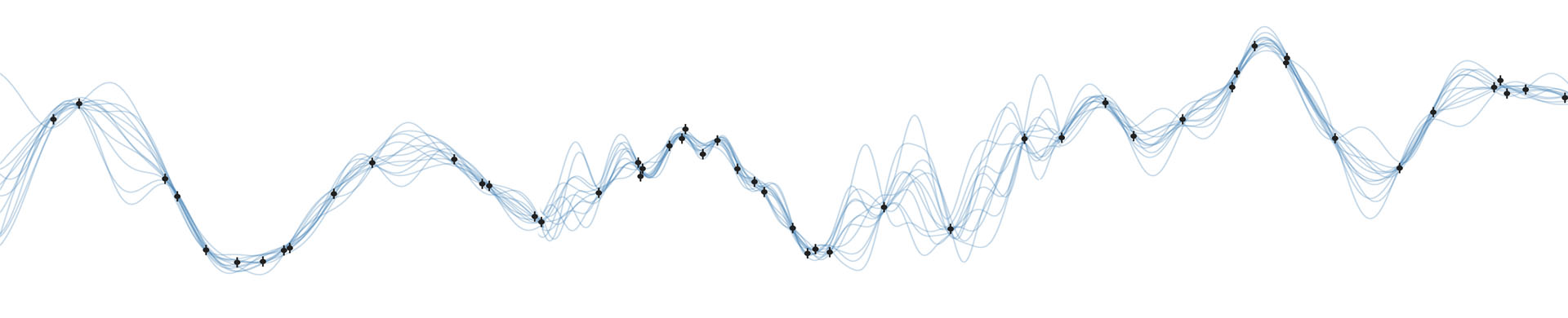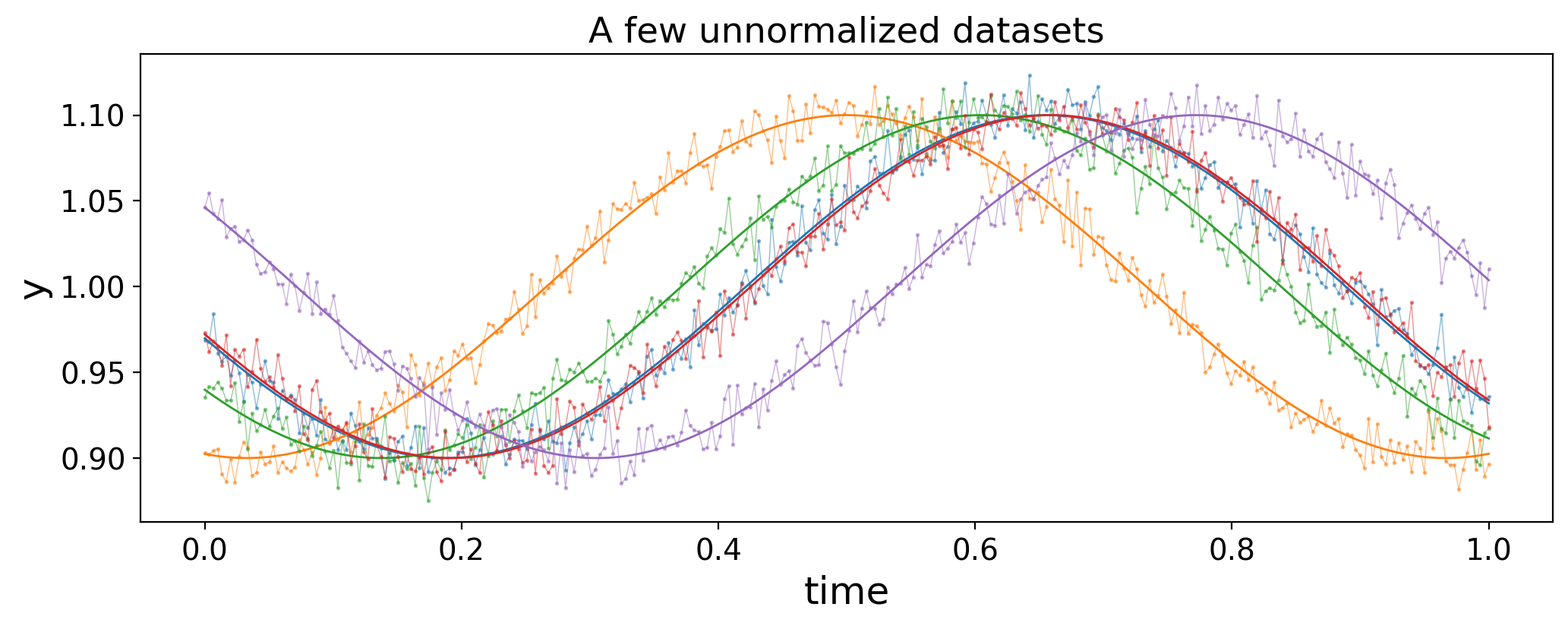### Normalized GPs

##### Config¶
In :
%matplotlib inline

In :
%config InlineBackend.figure_format = "retina"

In :
import matplotlib
import matplotlib.pyplot as plt
import numpy as np

# Disable annoying font warnings
matplotlib.font_manager._log.setLevel(50)

# Disable theano deprecation warnings
import warnings
warnings.filterwarnings('ignore', category=DeprecationWarning)
warnings.filterwarnings('ignore', category=FutureWarning)
warnings.filterwarnings('ignore', category=UserWarning, module='theano')

# Style
plt.style.use('default')
plt.rcParams['savefig.dpi'] = 100
plt.rcParams['figure.dpi'] = 100
plt.rcParams['figure.figsize'] = (12, 4)
plt.rcParams['font.size'] = 14
plt.rcParams['text.usetex'] = False
plt.rcParams['font.family'] = 'sans-serif'
plt.rcParams['font.sans-serif'] = ['Liberation Sans']
plt.rcParams['font.cursive'] = ['Liberation Sans']
plt.rcParams['mathtext.fontset'] = 'cm'
plt.rcParams['mathtext.fallback_to_cm'] = True

In :
del matplotlib; del plt; del warnings

##### Main¶

I ran into an interesting statistics problem while working on my interpretable Gaussian process for stellar light curves (see this post). What happens to the covariance structure of a dataset when we normalize it to its own mean (or median)? This is something that happens a lot in the literature: astronomers love normalizing light curves to sweep the unknown baseline under the rug and convert their data in relative units. But it turns out that this is sometimes a dangerous thing to do, since it can significantly change the covariance structure of the data. In this post I'll show an example of this, how it can bias GP inference, and how to fix it.

This post was generated from this Jupyter notebook and this helper Python script.

# The premise¶

It is standard practice in astronomy to mean- or median- normalize datasets, since one is often more interested in deviations from some baseline than in the value of the baseline itself. This is the case, for example, in searches for transiting exoplanets or photometric studies of stellar variability, where the raw data consists of a timeseries of fluxes measured in counts on the detector. The absolute number of counts from a particular target is physically uninteresting, as it depends on a host of variables such as the distance to the target, the collecting area of the instrument, and the quantum efficiency of the detector. However, fractional deviations from the mean number of counts are physically meaningful, as they can encode information such as the size of the transiting planet or the size and contrast of star spots. Normalization by the mean (or median) allows one to analyze data in units of (say) parts per million rather than counts per second.

Another common practice in astronomy is to model one's data (or at least a component of one's data) as a Gaussian process (GP). GPs offer a convenient, flexible, and efficient way of modeling correlated data and have seen extensive use in both transiting exoplanet and stellar variability studies. In one dimension, a GP is fully described by a mean vector $\pmb{\mu}$ and a covariance matrix $\pmb{\Sigma}$, the latter of which encodes information about correlations and periodicities in the data that are in some cases related to physical parameters of interest (such as the rotation period of a star or the lifetime of star spots).

In this post, I'm going to show that these two common practices can be somewhat at odds with each other. Specifically, if a physical process that generates a dataset is distributed as a GP, the normalized process cannot be distributed as a GP. Provided certain conditions are met, the normalized process can be well approximated by a GP, albeit one with a different covariance matrix $\tilde{\pmb{\Sigma}}$ that is not simply a scalar multiple of the original covariance matrix. Moreover, if the original process is described by a stationary kernel (i.e., one in which covariances are independent of phase), the normalized process is not guaranteed to be.

For most applications, none of this is likely to make much of a difference, since GPs are often used to model nuisance signals; in that case, the optimal hyperparameters describing the GP covariance are physically uninteresting. However, in cases where one wishes to interpret the GP hyperparameters in a physical context (such as using a periodic GP kernel to infer stellar rotation rates), normalizing one's data to the mean or median value can impart (potentially significant) bias. This can usually be fixed in a very simple way: just fit for the baseline, even if you think you know what it should be!

This post is only the beginning of my musings on the topic. I'm (very slowly) writing a paper on this here, and I hope to go in more depth in future posts.

# Setup¶

To show the issue with normalizing data, let's generate a synthetic dataset. We're going to keep things simple and generate datasets from a sinusoidal model with a fixed amplitude and period but a random phase, plus some Gaussian noise with known variance. We can pretend each dataset is a light curve of a star $-$ a set of measurements of the star's flux over time. We will then "observe" these data and model it using a periodic Gaussian process, and check whether this GP serves as a good estimator (unbiased, well calibrated) for the period.

In order to gauge any bias in the estimate, we need to generate many datasets. Again, they will all be generated from sinusoids with the same amplitude and period, but with random phases and random Gaussian noise.

In :
import numpy as np
import matplotlib.pyplot as plt
import george
from george.kernels import CosineKernel
import scipy.optimize as op
from tqdm.auto import tqdm
from scipy.stats import norm
import normgp
import theano
import theano.tensor as tt
from IPython.display import display, Markdown


Here are the settings we'll adopt for this experiment:

In :
npts = 300
ndatasets = 1000
mean = 1.0
sigma = 0.01
tmax = 1.0
amp_true = 0.1
p_true = 0.93427
t = np.linspace(0, tmax, npts)

In :
display(Markdown(f"""
| setting | description | value |
| - | :- | :-:
| npts | number of points per dataset | {npts} |
| ndatasets | number of datasets | {ndatasets}|
| mean | mean of the generating process | {mean} |
| sigma | uncertainty on each data point | {sigma} |
| tmax | independent variable runs from 0 to this value | {tmax} |
| amp_true | true amplitude of the process | {amp_true} |
| p_true | true period of the process | {p_true} |
"""))

setting description value
npts number of points per dataset 300
ndatasets number of datasets 1000
mean mean of the generating process 1.0
sigma uncertainty on each data point 0.01
tmax independent variable runs from 0 to this value 1.0
amp_true true amplitude of the process 0.1
p_true true period of the process 0.93427

Note that the period is slightly less than the baseline over which we're making the measurements, meaning that in each dataset we're observing slightly more than one period. As we will see, this is precisely the scenario in which GP inference on normalized data can lead to bias.

# Standard data¶

First, let's run our experiment on the actual raw data, meaning we won't do any normalization. (So this better work!)

## Generate the data¶

Let's generate ndatasets datasets with different realizations of the noise. Each dataset is simply a sine curve with amplitude amp_true, period p_true, and a random phase, plus Gaussian noise with standard deviation sigma.

In :
np.random.seed(0)
y_true = mean + amp_true * np.sin(
2 * np.pi / p_true * t.reshape(1, -1) + 2 * np.pi * np.random.random(size=(ndatasets, 1))
)
y = y_true + sigma * np.random.randn(ndatasets, npts)


We can plot a few of them to see what we're dealing with:

In :
for m in range(5):
plt.plot(t, y_true[m], lw=1, color="C{}".format(m))
plt.plot(t, y[m], lw=0.5, alpha=0.5, color="C{}".format(m))
plt.plot(t, y[m], ".", ms=2, alpha=0.5, color="C{}".format(m))
plt.xlabel("time", fontsize=18)
plt.ylabel("y", fontsize=18)
plt.title("A few unnormalized datasets");The thicker lines are the sine curves and the thinner lines (and points) are the noisy data we observe.

## Inference using a cosine kernel¶

Our task now is to infer the period of the sinusoid from each dataset using a periodic Gaussian process. We'll use the perfectly periodic CosineKernel and its implementation in the george package to compute the likelihood. Since we're going to run inference on a bunch of datasets, we're going to cut some corners and just optimize the parameters to find the maximum likelihood period and amplitude. We will then estimate the posterior variance as the negative reciprocal of the second derivative of the log-likelihood at this value (this is called the Laplace approximation). Note that we also have to optimize the amplitude of the GP, but for simplicity we'll just fix that at the maximum likelihood value when computing the posterior uncertainty on the period. (If you're concerned about any of this, see below: this turns out to be a very good approximation to the posterior for this particular problem).

In :
p_mean_george = np.empty(ndatasets)
p_std_george = np.empty(ndatasets)

def nll(x, data):
"""Return the negative log likelihood of data conditioned on params x."""
gp.set_parameter_vector(x)
ll = gp.log_likelihood(data, quiet=True)
return -ll if np.isfinite(ll) else 1e25

"""Return the gradient of the negative log likelihood of data conditioned on params x."""
gp.set_parameter_vector(x)

# Initial guesses (log amp^2, log period)
guess = [np.log(amp_true ** 2 / 2), np.log(p_true)]

# Set up the periodic GP
gp = george.GP(
np.exp(guess) * CosineKernel(guess), white_noise=np.log(sigma ** 2), mean=mean
)
gp.compute(t)

# Run the optimizer on each dataset
for m in tqdm(range(ndatasets)):

# Find the period at max likelihood
results = op.minimize(nll, guess, jac=grad_nll, method="L-BFGS-B", args=(y[m],))
_, p_mean_george[m] = np.exp(results.x)

# Laplace approximation to get the local posterior std. dev.
eps = 1e-8 * grad_nll(results.x, y[m])
p_std_george[m] = np.sqrt(
(2 * eps)
/ (
- grad_nll(results.x - np.array([0.0, eps]), y[m])
)
)


Here is the distribution of maximum likelihood estimates of the period for the inference runs on each of our ndatasets datasets:

In :
plt.hist(p_mean_george, bins=20, density=True, label="estimates")
plt.axvline(np.mean(p_mean_george), color="k", ls="--", label="mean");
plt.axvline(p_true, color="C1", label="truth")
plt.xlabel("period", fontsize=18)
plt.ylabel("probability density", fontsize=18)
plt.legend()
plt.title("Period estimates using a GP");